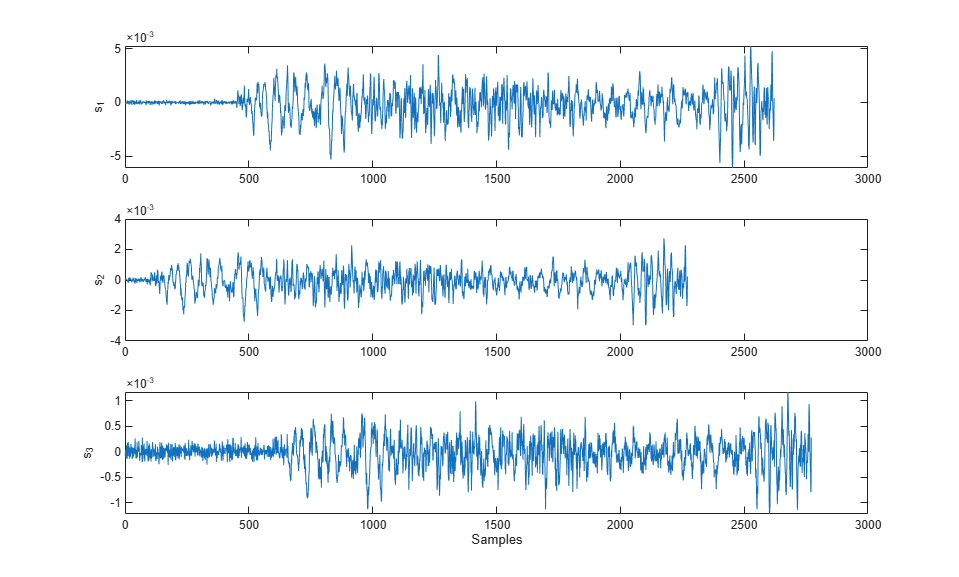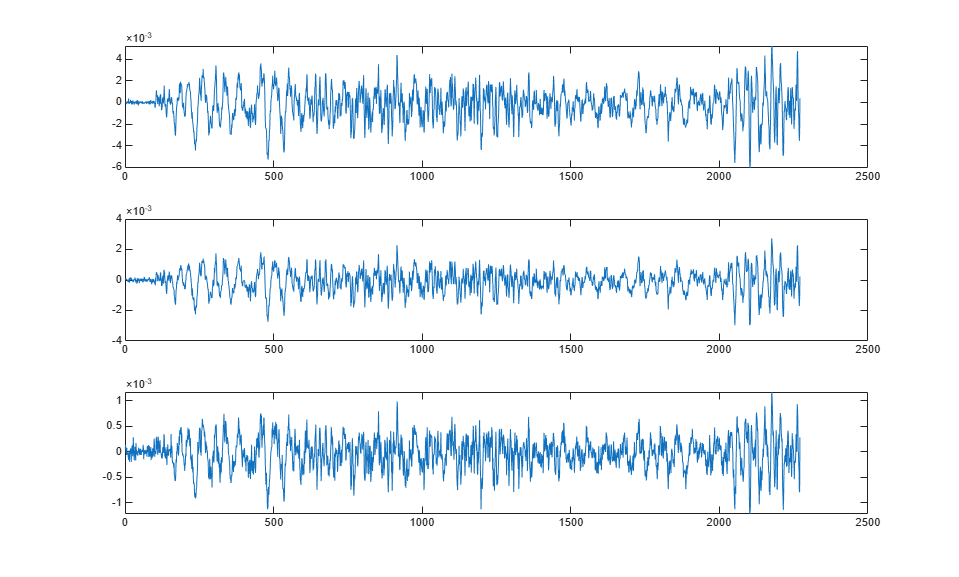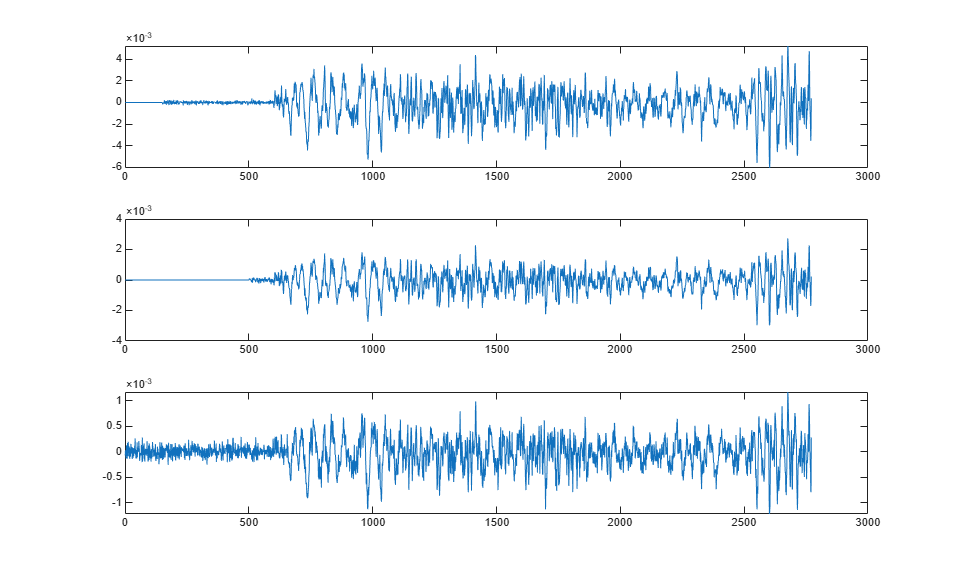# Align Signals with Different Start Times

Many measurements involve data collected asynchronously by multiple sensors. If you want to integrate the signals, you have to synchronize them. The Signal Processing Toolbox™ has functions that let you do just that.

For example, consider a car crossing a bridge. The vibrations it produces are measured by three identical sensors located at different spots. The signals have different arrival times.

Load the signals into the MATLAB® workspace and plot them.

```load relatedsig ax(1) = subplot(3,1,1); plot(s1) ylabel('s_1') ax(2) = subplot(3,1,2); plot(s2) ylabel('s_2') ax(3) = subplot(3,1,3); plot(s3) ylabel('s_3') xlabel('Samples') linkaxes(ax,'x') ```Signal `s1` lags `s2` and in turn leads `s3`. The delays can be computed exactly using `finddelay`. You see that `s2` leads `s1` by 350 samples, `s3` lags `s1` by 150 samples, and `s2` leads `s3` by 500 samples.

```t21 = finddelay(s2,s1) t31 = finddelay(s3,s1) t32 = finddelay(s2,s3) ```
```t21 = 350 t31 = -150 t32 = 500 ```

Line up the signals by leaving the earlier signal untouched and clipping the delays out of the other vectors. Add 1 to the lag differences to account for the one-based indexing used by MATLAB®. This method aligns the signals using as reference the earliest arrival time, that of `s2`.

```axes(ax(1)) plot(s1(t21+1:end)) axes(ax(2)) plot(s2) axes(ax(3)) plot(s3(t32+1:end)) ```Use `alignsignals` to align the signals. The function works by delaying earlier signals, so use as reference the latest arrival time, that of `s3`.

```[x1,x3] = alignsignals(s1,s3); x2 = alignsignals(s2,s3); axes(ax(1)) plot(x1) axes(ax(2)) plot(x2) axes(ax(3)) plot(x3) ```The signals are now synchronized and ready for further processing.